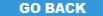Or:# Webmath

 Top Subjects:  Animals   Art/Architecture   Geography   Health   History   Math   Reading   ScienceResource:Webmath
Provider:Webmath
Summary:Age Range: 5-18 (Grades K-12, with parental supervision). Webmath solves your math problems instantly. Are you working on a math problem and just can't figure it out? Do need a fraction converted to a decimal quickly?
Description:Do you need to calculate compound interest on that loan? Having trouble with a long division problem? Do you want the definition of a "ratio"? Need help solving a word problem? Want to calculate right triangles? Do you want to find the square root of a number? Would you like help plotting conic sections? This site is for you!

As explained at the website: "Webmath is a math-help web site that generates answers to specific math questions and problems, as entered by a user, at any particular moment. The math answers are generated and displayed real-time, at the moment a web user types in their math problem and clicks 'solve.' In addition to the answers, Webmath also shows the student how to arrive at the answer."

For example, if the user wants to know how to square the quantity (x+2), Webmath does not just display the answer x2+4x+4, but a step-by-step solution as well.

How cool is that?

When you get to the site just choose the category for your particular math problem, fill it in, and let Webmath guide you to a solution. Categories include:
• Math for Everyone
• General Math
• K-8 Math
• Algebra
• Plots & Geometry
• Trig. & Calculus
• Other Stuff
Bookmark this site, as it not only helps with occasional math problems, but it's fun to explore. The curious will get all kinds of math practice without any drill work at all.
C A T E G O R I E S
Orientation:General
Subjects:Math
Teaching Methods:Online Interactive
I N T E N D E D   F O R:
Ages:5,6,7,8,9,10,11,12,13,14,15,16,17,18